# Electronic Circuit Simulation - Electromagnetism Background (part 2) [Python][Custom Thumbnail]

All the Code of the series can be found at the Github repository:
https://github.com/drifter1/circuitsim

# Introduction

Hello it's a me again @drifter1! Today we continue with the Electric Circuit Simulation series, a tutorial series where we will be implementing a full-on electronic circuit simulator (like SPICE) studying the whole concept and mainly physics behind it! In this article we will cover Electronic Circuits and more specifically:
• Resistor Combinations (Series, Parallel and Mixed)
• Current and Voltage Dividers
• Electronic Circuit Terminology
• Kirchhoff's Laws
• Electric Components (Active and Passive)
• Capacitors and Inductors

## Requirements:

• Physics and more specifically Electromagnetism Knowledge
• Knowing how to solve Linear Systems using Linear Algebra
• Some understanding of the Programming Language Python

## Difficulty:

Talking about the series in general this series can be rated:
Today's topic(s) can be rated:
• Basic

# Actual Tutorial Content

## Resistor Combinations

Resistors can be combined together forming networks that are known as Resistor Combinations. Instead of having a dozen Resistors connected to each other in series, parallel or even mixed, we tend to calculate the so called Equivalent Resistance of them, which replaces many Resistors with a single one! To do that easily we think of the circuit as parts which have Resistors connected "in series" or "in parallel" and solve the whole problem in specific steps. So, let's get into these two cases and how we solve them, and also how we solve Mixed Problems...

### Series

Consider Resistors where Current flows through them sequentially, which means that all of them have the same Current, like in the following diagram:[Image 1]

The sum of the voltages of each resistor in such a combination is equal to the total voltage given by the voltage supply they are connected to. Therefore using Ohm's Law, we easily get the following equation for Equivalent Resistance:### Parallel

Consider Resistors that are connected directly to the same voltage source, meaning that they have the same Voltage, like in the following diagram:[Image 2]

In this case, the Current will split, making the total sum of the individual Current through each Resistor equal to the total "supplied" current. Using Ohm'w law again we end up with the following equation for the Equivalent Resistance: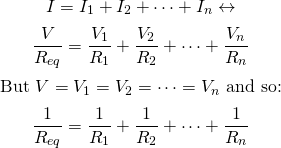### Mixed

From the previous two cases we know that:
• Series Resistors have the same Current
• Parallel Resistors have the same Voltage
In a mixed situation we just have to find these two types of connections and to substitute them with their individual equivalent resistance. For example think about the following diagram:[Image 3]

Here R2 and R3 are connected in Parallel, whilst R1 is connected in Series to each of them. So, we first have to calculate the equivalent Resistance of R2 and R3, let's say R2,3, and then the equivalent resistance of R1 and R2,3, lets' say R1,2,3 or Req. In equations this looks like this: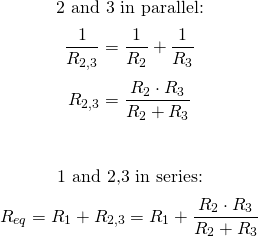For Resistor Combinations you can also check out my article:
Physics - Electromagnetism - Resistor Combinations

These equations will be quite useful in our simulator as we will be able to calculate equivalent resistances, as the impact of lots of Resistors in an electronic circuit can be measured by one Resistor that substitutes the others with an Equivalent Resistance...

## Current and Voltage Dividers

Two quite useful circuits in Electronics are the so called Current and Voltage Dividers. As their name suggests, the first gives us the ability to divide the total current into fractional parts (think about Resistors in parallel), whilst the second gives us the ability to divide the total voltage into fractional parts (think about Resistors in series). Using specific formulas, someone can calculate the Current and Voltage respectively for specific parts of an circuits where such combinations occur. So, let's now get into how each of them and which Formula can be used to determine the Current and Voltage correspondingly...

### Current Divider

Suppose that we have some Resistors connected in Parallel. The current through the Resistor is equal to the voltage applied to it, which is also the same for all Resistors, divided by its Resistance. Thinking about the Equivalent Resistance Rtotal, the voltage can also be written as a product between the total current times Rtotal. So, the current that flows through any resistor n is: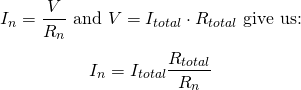The Current Divider Formula

### Voltage Divider

Let's now suppose that we have some Resistors connected in Series. The voltage of each resistor is equal to the product of the current that flows through it, which is also the same for all of the resistors, times its Resistance. Knowing that the current is the same for all resistors, it's also the same when thinking about the Equivalent Resistance, and so we can write the total current as the division between the total voltage and equivalent Resistance. So, in the end we get:The Voltage Divider Formula

These two equations can also become quite handy as we will be able to calculate the current and voltage respectively, knowing only the resistances and total current and voltage respectively!

## Electronic Circuit Terminology

To understand the various terms that we will be using in our Electronic Circuit Analysis, we should give a small definition for the most important of them:
• Branch: A branch represents a single circuit element like a voltage source or a resistor and so any two-terminal element.
• Node: A node is the point of connection between two or more branches, and so circuit elements.
• Junction: A junction is the point where different wires are interconnected, which means that nodes could be made up of more than one junctions!
• Loop: A loop is any closed path in a circuit.
• Mesh: A mesh is a loop with no other loops inside it.

For example let's suppose that we have the following circuit schematic: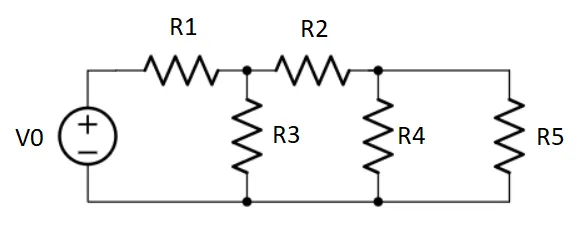[Image 4]

These circuit has:
• 6 branches, cause there are five resistors and one voltage source, giving a total of 5 + 1 = 6 circuit elements
• 4 nodes, connecting V0-R1, R1-R2-R3, R2-R4-R5 and V0-R3-R4-R5 correspondingly
• 6 loops, the outer loop (V0-R1-R2-R5), three small loops (V0-R1-R3, R3-R2-R4, R4-R5) and two larger loops (V0-R1-R2-R4, R3-R2-R5)
• 3 meshes, which are the three smaller loops (V0-R1-R3, R3-R2-R4, R4-R5)

Understanding this terminology will be very important in the Mesh and Nodal Analysis that we will be starting from next time...

## Kirchhoff's Laws

Anyone who did Electromagnetism in school or university has learned about Kirchhoff's Laws alongside Ohm's law. Let's refresh your knowledge about them!

### KCL

Kirchhoff's Current Law (KCL) tells us that the total current or charge entering a junction or node is equal to the charge leaving the node, cause the charge has no other place to go except to leave as no charge is lost within the node (conservation of charge). Mathematically this can be represented as: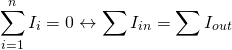### KVL

Kirchhoff's Voltage Law (KVL) tells us that the total voltage around a loop is zero and equal to the sum of all the voltage drops within the same loop. Mathematically we have: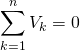To remember how we solve problems using KCL and KVL you can read my article:
Physics - Electromagnetism - Kirchhoff's laws with applications

## Electric Components

Electric Components are split into two different categories:
1. Active: Components which produce energy in form of Voltage or Current (e.g. independent voltage or current sources)
2. Passive: Components which store or convert Energy in form of Voltage and Current (e.g. resistors, capacitors, inductors that convert into thermal, electric and magnetic energy correspondingly)

## Capacitors and Inductors

Capacitors and Inductors are two very important circuit elements for Transient Analysis mostly, as their behavior is quite unique. I'm not sure if we will get into TA, in the moment, but why not give some basic knowledge about them?

### Capacitor

A Capacitor is characterized by its property known as Capacitance, symbolized with C and calculated in Farad (F). This quantity is given by the equation: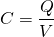Thinking about current, current is equal to the rate of change of charge over time, meaning that we can end up with the following Current-Voltage Relationship:
The voltage can be given by the following integral: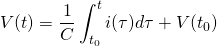### Inductor

An Inductor is characterized by it's property known as Inductance, symbolized by L and calculated in Henry (H). For a coil this quantity is given by the equation: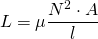where:
• μ is is the magnetic permeability of the coil
• N the number of turns of a coil
• A the area of the coil
• l the length of the coil
From Electromagnetic Induction we know that the Induced Voltage is given by: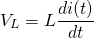Using this we can also calculate the current as an integral: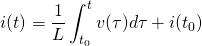For more information around Capacitors you can also check out my articles:
Physics - Electromagnetism - Capacitors (Condensers) and Capacitance
Physics - Electromagnetism - How to solve problems around Capacitors

In the same way, for Inductors you can also check out:
Physics - Electromagnetism - Self Induction

## RESOURCES

### References:

1. https://www.electronics-tutorials.ws/resistor/res_5.html
4. https://electrical-engineering-portal.com/electric-circuits-nodes-branches-loops
6. https://isaacphysics.org/concepts/cp_kirchhoffs_laws
7. https://www.electricaltechnology.org/2013/06/the-main-difference-between-active-and.html
8. https://www.codrey.com/dc-circuits/active-and-passive-elements/
9. https://www.electronics-tutorials.ws/capacitor/cap_1.html
10. https://www.electronics-tutorials.ws/inductor/inductor.html

### Images:

1.2.3.Mathematical Equations were made using quicklatex

## Final words | Next up on the project

And this is actually it for today's post! I hope that I helped you refresh your knowledge about Electronic Circuits!

Next up on this series are the solving methods: Mesh and Nodal Analysis.

So, see ya next time!

## GitHub Account:

https://github.com/drifter1Keep on drifting! ;)

H2
H3
H4
3 columns
2 columns
1 column